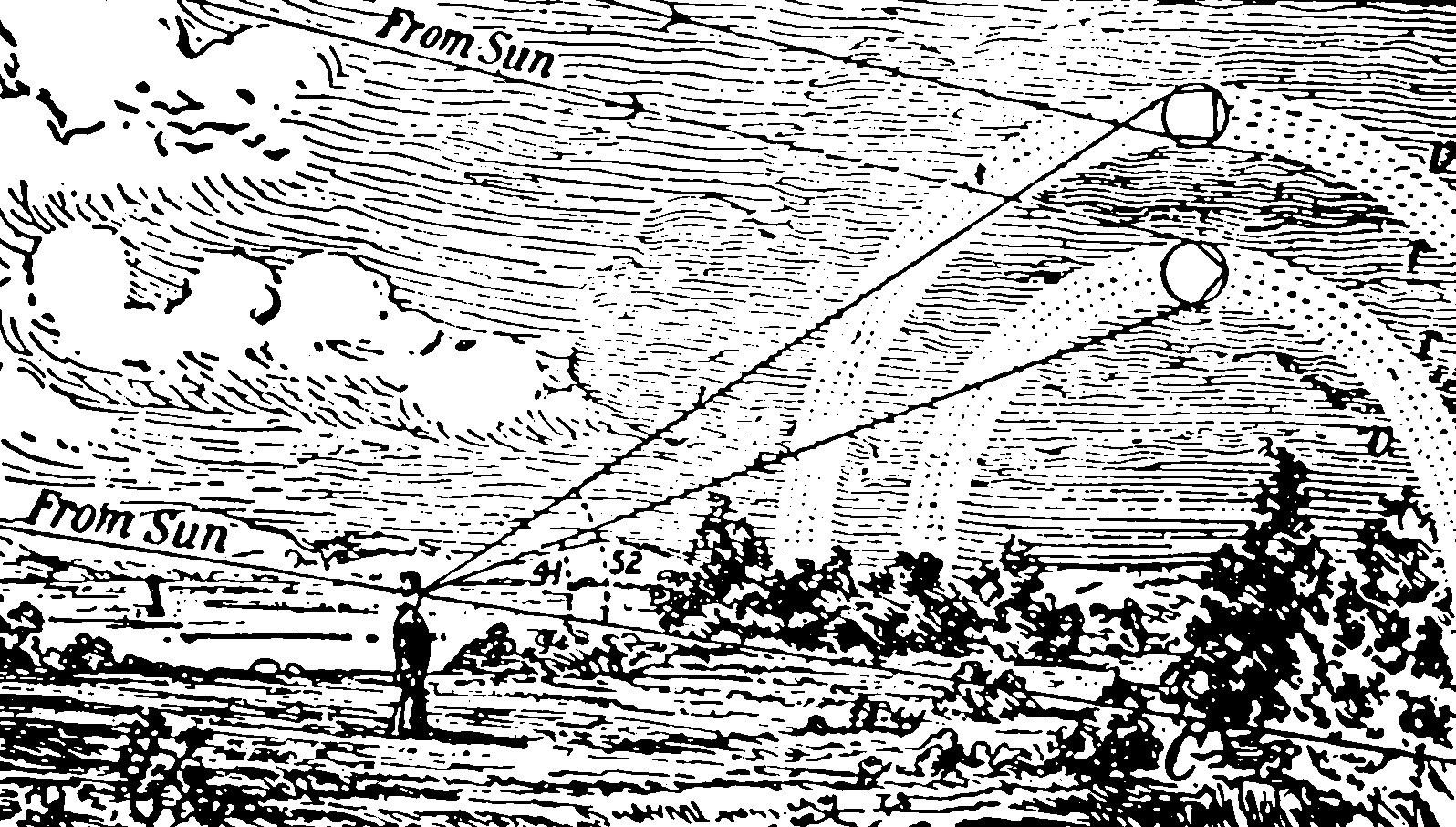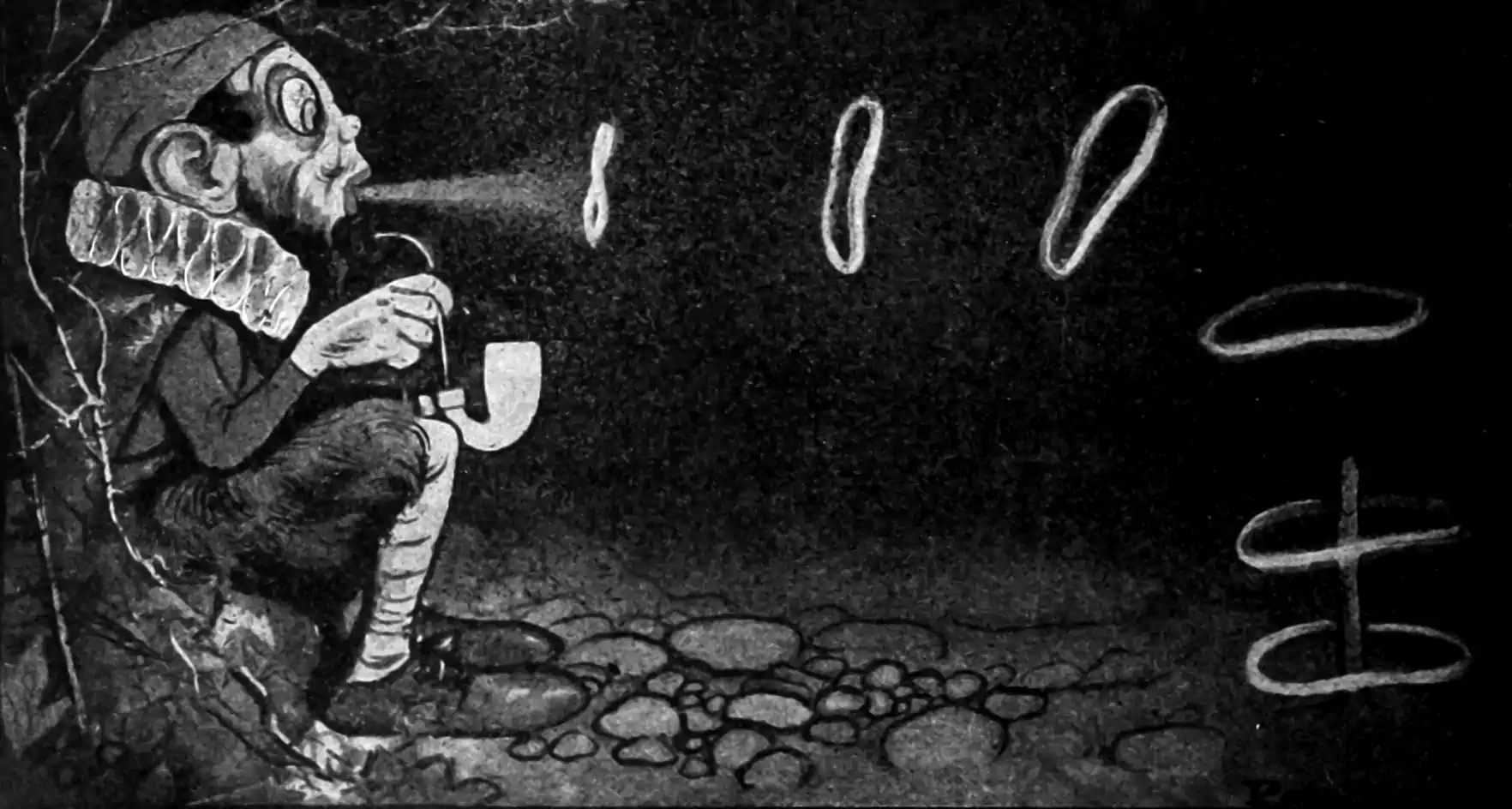# Wiener-Khintchine representations

## Spectral representations of stochastic processes$\renewcommand{\var}{\operatorname{Var}} \renewcommand{\Ex}{\mathbb{E}} \renewcommand{\Pr}{\mathbb{P}} \renewcommand{\dd}{\mathrm{d}} \renewcommand{\pd}{\partial} \renewcommand{\vv}{\boldsymbol{#1}} \renewcommand{\mmm}{\mathrm{#1}} \renewcommand{\cc}{\mathcal{#1}} \renewcommand{\ff}{\mathfrak{#1}} \renewcommand{\oo}{\operatorname{#1}} \renewcommand{\gvn}{\mid} \renewcommand{\rv}{\mathsf{#1}}$

Consider a real-valued stochastic process $$\{\rv{f}_{\vv{t}}\}_{\vv{t}\in\mathcal{T}}$$ such that a realisation of such process is a function $$\mathcal{T}\to\mathbb{R}$$ where $$\mathcal{T}\subseteq \mathcal{R}^d$$ is some compact set of non-zero Lebesgue volume, like a hypercube, or all of $$\mathbb{R}^{d}$$.1 We call $$\mathcal{T}$$ the index.

Suppose the process is described by a probability measure $$\mu_{\vv{t}}, \vv{t}\in\mathcal{T}$$ such that for $$\vv{t},\vv{s}\in\mathcal{T}$$, the process has expectation function $\Ex[t]=\Ex[\rv{f}_{\vv{t}}]=\int_{\mathbb{R}} x \mu_{\vv{t}}(\dd x)$ and covariance \begin{aligned} K(\vv{t}, \vv{s}) &=\operatorname{Cov}\left\{\rv{f}_{\vv{t}}, \rv{f}_{\vv{s}}\right\}\\ &=\Ex[\rv{f}_{\vv{t}} \rv{f}_{\vv{s}}]-\Ex[\rv{f}_{\vv{t}}]\Ex[ \rv{f}_{\vv{s}}] \\ &=\iint_{\mathbb{R}^{2}} \vv{s}\vv{t} \mu_{\vv{t}, \vv{s}}(\dd \vv{s} \times \dd \vv{t})-\Ex[\rv{f}_{\vv{t}}]\Ex[ \rv{f}_{\vv{s}}] \end{aligned} We are concerned with ways to represent this covariance function $$K$$.

I do not want to arse about with this mean function overmuch since it only clutters things up, so hereafter we will assume $$\Ex[\vv{t}]=0$$ unless stated otherwise.

## Wiener theorem: Deterministic case

This is also interesting and I wrote it up for a different project: See Wiener theorem.

## Wiener-Khinchine theorem: Spectral density of covariance kernels

I found the Wikipedia introduction usually confusing. I recommend a well-written article, e.g. Abrahamsen (1997) or Robert J. Adler, Taylor, and Worsley (2016). Anyway, this theorem governs wide-sense-stationary random processes. Here wide-sense-stationary, a.k.a. weakly stationary or sometimes homogeneous, requires that

1. the process mean function is constant, $$\Ex[\vv{t}]=0,$$ w.l.o.g. and
2. correlation depends only on $$\vv{t}-\vv{s}$$, i.e. $$K(\vv{t}, \vv{s})=K(\vv{t}-\vv{s}).$$

That is, the first two moments of the process are stationary, but other moments might do something weird. For the wildly popular case of Gaussian processes, since the first two moments uniquely determine the process, these end up being the same. In this context, the Wiener-Khintchine theorem tells us that there exists a finite positive measure $$\nu$$ on the Borel subsets of $$\mathbb{R}^d$$ such that the covariance kernel is given $K(\vv{\tau} )=\int \exp(2\pi i\vv{\omega}^{\top}\tau )\nu(\dd \vv{\omega}).$

If $$\nu$$ has a density $$\psi(\vv{\omega})$$ with respect to the dominating Lebesgue measure, then $\psi(\vv{\omega})=\int K(\vv{\tau} )\exp(-2\pi i \vv{\omega}^{\top} \vv{\tau} )\,\dd\vv{\tau}.$ That is, the power spectral density and the covariance kernel are Fourier dual. Nifty.

What does this mean? Why do I care? Turns out this is useful for many reasons. It relates the power spectral density to the correlation function, and also to continuity/differentiability.

## Bochner’s Theorem: stationary spectral kernels

Everyone seems to like the exposition in Yaglom (1987), which I brusquely summarise here. Bochner’s theorem tells us that $$K:\mathcal{T}\to\mathbb{R}$$ is the covariance function of a weakly stationary, mean-square-continuous, complex-valued random process on $$\mathbb{R}^{d}$$ if and only if it can be represented as $K(\vv{\tau})=\int_{\mathcal{T}} \exp \left(2 \pi i \vv{\omega}^{\top} \vv{\tau}\right) \nu(\mathrm{d} \vv{\omega})$ where $$\nu$$ is a positive and finite measure on (the Borel subsets of) $$\mathbb{C}^d.$$ If $$\nu$$ has a density $$\psi(\vv{\omega})$$ with respect to the dominating Lebesgue measure, then $$\psi$$ is called the spectral density of $$K,$$ and $$\psi$$ and $$K$$ are Fourier duals. This is what Robert J. Adler, Taylor, and Worsley (2016) calls the spectral distribution theorem.

This looks similar to the Wiener-Khintchine theorem, no? This one is telling us that the power spectrum represents all possible stationary kernels, i.e. we are not missing out on any by using a spectral representation. Note also that we needed to generalize this to complex-valued fields, and consider integrals over complex indices for it to make sense; the real fields arise as a special case.

## Yaglom’s theoremSome of the kernel design literature cites a generalised Bochner-type Theorem , Yaglom’s Theorem, which does not presume stationarity:

A complex-valued, bounded, continuous function $$K$$ on $$\mathbb{R}^{d}$$ is the covariance function of a mean-square-continuous, complex-valued, random process on $$\mathbb{R}^{d}$$ if and only if it can be represented as $K(\vv{s}, \vv{t})=\int_{\mathcal{T} \times\mathcal{T}} e^{2 \pi i\left(\vv{\omega}_{1}^{\top} \vv{s}-\vv{\omega}_{2}^{\top} \vv{t}\right)} \nu\left(\dd \vv{\omega}_{1}\times \dd \vv{\omega}_{2}\right).$

This is reassuring, but does not constrain kernel designs in an obviously useful way to my tiny monkey brain.

## Spectral representation

This is nearly simple, but has the minor complication that the most intuitive route (to my mind at least) requires us to admit complex stochastic processes.

TBD; mention power spectrum, spectral moments.

1. We can take it to be a sub manifold but things get more subtle and complex.↩︎

### No comments yet. Why not leave one?

GitHub-flavored Markdown & a sane subset of HTML is supported.